• ## Six equalizers for V-BLAST

In the past, we had discussed several posts on two transmit two receive MIMO communication, where the transmission was based on V-BLAST. The details about V-BLAST can be read from the landmark paper V-BLAST: An [...]

• ## Transmit beamforming

In this post lets discuss a closed-loop transmit diversity scheme, where the transmitter has the knowledge of the channel. As there is a feedback path required from the receiver, to communicate the channel seen by [...]

• ## Alamouti STBC with 2 receive antenna

In the past, we had discussed two transmit, one receive antenna Alamouti Space Time Block Coding (STBC) scheme. In this post, lets us discuss the impact of having two antennas at the receiver. For the [...]

• ## MIMO with ML equalization

We have discussed quite a few receiver structures for a 2×2 MIMO channel namely, (a) Zero Forcing (ZF) equalization (b) Minimum Mean Square Error (MMSE) equalization (c) Zero Forcing equalization with Successive Interference Cancellation (ZF-SIC) [...]

• ## MIMO with MMSE SIC and optimal ordering

This post attempts to build further on the MIMO equalization schemes which we have discussed – (a) Minimum Mean Square Error (MMSE) equalization, (b) Zero Forcing equalization with Successive Interference Cancellation (ZF-SIC) and (c) ZF-SIC [...]

• ## MIMO with ZF SIC and optimal ordering

In previous posts, we had discussed equalization of a 2×2 MIMO channel with Zero Forcing (ZF) equalization and later, Zero Forcing equalization with successive interference cancellation (ZF-SIC). In this post, we will explore a variant [...]

• ## MIMO with Zero Forcing Successive Interference Cancellation equalizer

The post on MIMO with Zero Forcing equalizer discussed a probable way of equalizing a 2×2 MIMO channel. The simulated results with the 2×2 MIMO system with zero forcing equalizer showed matching results as obtained [...]

• ## MIMO with MMSE equalizer

In a previous post, we had discussed a 2×2 MIMO transmission using BPSK modulation in Rayleigh channel with a Zero Forcing equalizer. The simulated results with the 2×2 MIMO system  with zero forcing equalizer showed [...]

• ## MIMO with Zero Forcing equalizer

We had discussed three Single Input Multiple Output (SIMO also known as receive diversity) schemes – Selection combining, Equal Gain Combining, Maximal Ratio Combining and a Multiple Input Single Output (MISO, also known as transmit [...]

• ## Alamouti STBC

In the recent past, we have discussed three receive diversity schemes – Selection combining, Equal Gain Combining and Maximal Ratio Combining. All the three approaches used the antenna array at the receiver to improve the [...]

## OFDM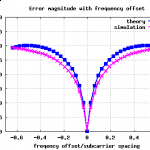Inter Carrier Interference (ICI) in OFDM due to frequency offset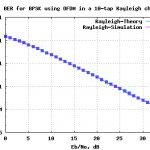BER for BPSK in OFDM with Rayleigh multipath channel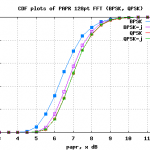Trying out PAPR reduction for OFDM by multiplication with j

## MIMO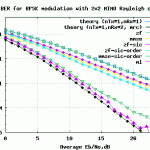Six equalizers for V-BLAST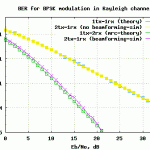Transmit beamforming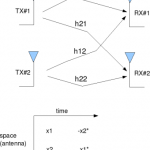Alamouti STBC with 2 receive antenna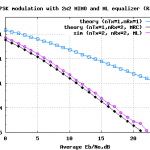MIMO with ML equalization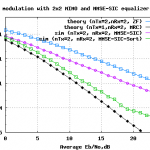MIMO with MMSE SIC and optimal ordering

## AnalogImage Rejection Ratio (IMRR) with transmit IQ gain/phase imbalance

The post on IQ imbalance in transmitter, briefly discussed the effect of amplitude and phase imbalance and also showed that IQ imbalance results in spectrum at the image frequency. In this article, we will quantify [...]

## Modulation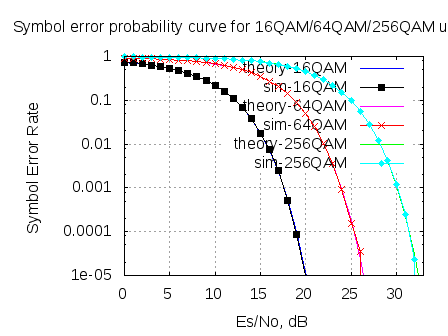Symbol Error rate for QAM (16, 64, 256,.., M-QAM)

In May 2008, we derived the theoretical symbol error rate for a general M-QAM modulation (in  Embedded.com, DSPDesignLine.com and dsplog.com) under Additive White Gaussian Noise. While re-reading that post, felt that the article is nice and warrants a [...]

## Channel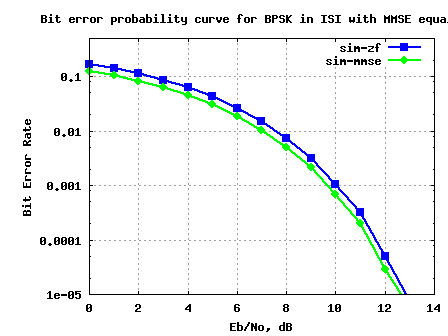BER for BPSK in ISI channel with MMSE equalization

In the past, we had discussed BER for BPSK in flat fading Rayleigh channel and BER for BPSK in a frequency selective channel using Zero Forcing Equalization. In this post, lets discuss a frequency selective [...]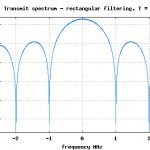BER with Matched FilteringMIMO with ML equalization

Solved!

Back!

## Coding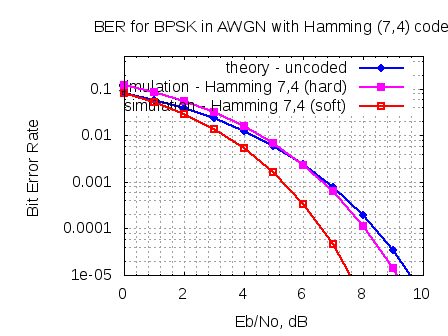Hamming (7,4) code with soft and hard decoding

An earlier post we discussed hard decision decoding for a Hamming (7,4) code and simulated the the bit error rate. In this post, let us focus on the soft decision decoding for the Hamming (7,4) [...]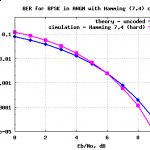Hamming (7,4) code with hard decision decoding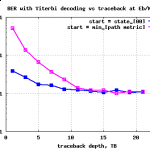Viterbi with finite survivor state memory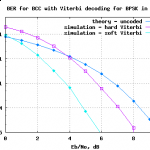Soft Input Viterbi decoder

## GATE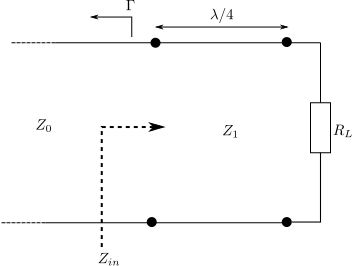GATE-2012 ECE Q28 (electromagnetics)

Question 28 on electromagnetics from GATE (Graduate Aptitude Test in Engineering) 2012 Electronics and Communication Engineering paper. Q28. A transmission line with a characteristic impedance of 100 is used to match a 50 section to a 200 section. If the matching [...]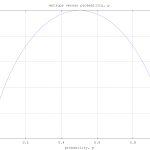GATE-2012 ECE Q15 (communication)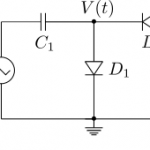GATE-2012 ECE Q13 (circuits)